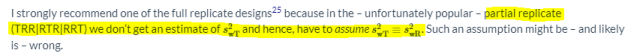WinnieH
☆

Sweden,
2023-08-18 10:57
(39 d 19:32 ago)

Posting: # 23697
Views: 591

## homoscedasticity assumption in RSABE method [RSABE / ABEL]

Dear all,

I had a question related to the homoscedasticity assumption in the RSABE method when I read the tutorial of PowerTOST.
PowerTOST-RSABE

If the RSABE method is performed on the highly variable drug with the 4-way crossover design (not the NTI drug), is the homoscedasticity assumption always holden? And I think if a 3-way crossover design is used, there is no way to assume the homoscedasticity since the test drug is administered once and we can not calculate the WSV of the test drug.

A further question is, when do we assume the reference and test product have equal variances, i.e. CVwT ≡ CVwR? And why?

Winnie

Helmut
★★★Vienna, Austria,
2023-08-18 13:23
(39 d 17:06 ago)

@ WinnieH
Posting: # 23698
Views: 462

## heteroscedasticity in RSABE (and ABEL)

Hi Winnie,

❝ If the RSABE method is performed on the highly variable drug with the 4-way crossover design (not the NTI drug), is the homoscedasticity assumption always holden?

It’s an assumption. In many cases it was proven outright false (quite often CVwT < CVwR). In RSABE (and ABEL as well) regulators are not interested in CVwT.

❝ And I think if a 3-way crossover design is used, there is no way to assume the homoscedasticity since the test drug is administered once and we can not calculate the WSV of the test drug.

You are right for the partial replicate TRR|RTR|RRT. No problems in the 3-period full replicates TRT|RTR or TRR|RTT.

❝ A further question is, when do we assume the reference and test product have equal variances, i.e. CVwT ≡ CVwR? And why?

If we have only information from a pilot study in a partial replicate design that’s all we can do.
If the true CVwT < CVwR, the study will be overpowered, which is economically and ethically questionable. If it will be the other way around, bad luck. See also this article about heteroscedasticity in RSABE and that one for ABEL.

Dif-tor heh smusma 🖖🏼 Довге життя Україна!Helmut SchützThe quality of responses received is directly proportional to the quality of the question asked. 🚮
Science Quotes
WinnieH
☆

Sweden,
2023-08-18 15:05
(39 d 15:24 ago)

@ Helmut
Posting: # 23700
Views: 453

## heteroscedasticity in RSABE (and ABEL)

Thank you so much for the answer and the references. I am looking through them now.

Best regards,
Zhe

BEQool
☆

Slovenia,
2023-08-18 14:30
(39 d 16:00 ago)

@ WinnieH
Posting: # 23699
Views: 454

## homoscedasticity assumption in RSABE method

❝ I had a question related to the homoscedasticity assumption in the RSABE method when I read the tutorial of PowerTOST.

❝ If the RSABE method is performed on the highly variable drug with the 4-way crossover design (not the NTI drug), is the homoscedasticity assumption always holden? And I think if a 3-way crossover design is used, there is no way to assume the homoscedasticity since the test drug is administered once and we can not calculate the WSV of the test drug.

Hello all,

I also have a similar question regarding calculation of s2wT (mentioned in this article about SABE based on the CVw of a Crossover Design). In the article the following equations are stated:And at the end this is written:So my question would be, why cant we get (calculate) s2wT from the partial replicate design? We obviously get s2wR and dont we also get s2w ? So then from the equation marked in yellow in the first picture, we can get s2wT by: (2* s2w - s2wR )? Or am I missing something here?

Best regards
BEQool
d_labes
★★★

Berlin, Germany,
2023-08-18 16:11
(39 d 14:18 ago)

@ BEQool
Posting: # 23701
Views: 441

❝ So my question would be, why cant we get (calculate) s2wT from the partial replicate design? We obviously get s2wR and dont we also get s2w ? So then from the equation marked in yellow in the first picture, we can get s2wT by: (2* s2w - s2wR )? Or am I missing something here?

Dear BEQool,
simply have a look at the sentences following your citation of the formulas in the article about SABE based on the CVw of a Crossover Design)

Regards,

Detlew
BEQool
☆

Slovenia,
2023-08-18 19:16
(39 d 11:13 ago)

@ d_labes
Posting: # 23702
Views: 419

Dear Detlew,
thank you for the answer. I had read the whole article but I am still not getting itPartial replicate design is a crossover design so we can get S2W (and consequently CVW) and because R is replicated we can also get S2WR (and consequently CVWR).
So why cant we get S2WT (and consequently CVWT) from the following equation:
S2W=(S2WT+S2WR)*2
as we only have one unknown (S2WT)?

"There is an infinite number of combinations of CVwT- and CVwR- values giving the same pooled CVw. We simply don’t – and can’t – know the variance components.
It’s like asking a pupil “We added two numbers and their sum was five. What were the two numbers?” Leaves the pupil – rightly – dazed and confused."

--> Refering to this, if we know that the sum (S2W) is 5 and one other number (S2WR; lets say it is 3), we can get the unknown number (S2WT; in this case we get 2)?

BEQoolIng. Helmut Schütz